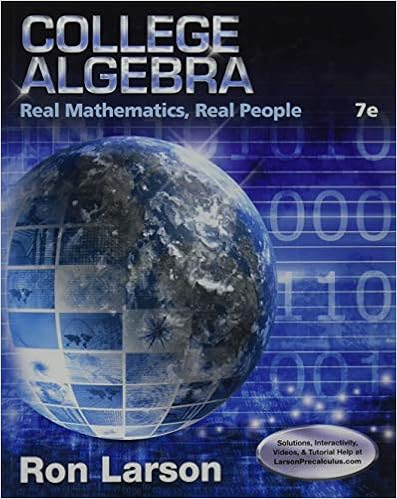# In some cases, it may be more realistic to consider a...

• Homework Help
• 2
• 100% (4) 4 out of 4 people found this document helpful

This preview shows page 1 - 2 out of 2 pages.

##### We have textbook solutions for you!
The document you are viewing contains questions related to this textbook.The document you are viewing contains questions related to this textbook.
Chapter P / Exercise 114
College Algebra: Real Mathematics, Real People
LarsonExpert Verified
Stat 331 - Assignment 1 - Winter 2012 Due on Friday June 1st by 5pm in my office (M3 3112). 1. In some cases, it may be more realistic to consider a simple linear regression model through the origin: y i = βx i + i , i = 1 , 2 , . . . , n where i iid N (0 , σ 2 ) (a) Find an expression for the least squares estimate of β and denote it as ˆ β . (b) Show that ˆ β is an unbiased estimator of β and find an expression for V ( ˆ β ) . (c) Let e i = y i - ˆ βx i denote the estimated residual. i. Show that n i =1 e i x i = 0 . ii. Is it true that n i =1 e i = 0 ? iii. Hence, can you conclude that sample correlation between the estimated resid- uals and the predictor variable equals zero? That is, do we have n i =1 ( e i - ¯ e )( x i - ¯ x ) p n i =1 ( e i - ¯ e ) 2 p n i =1 ( x i - ¯ x ) 2 = 0? (d) Provide an expression for the least squares estimate of σ 2 . 2. Consider the simple linear regression model: y i = β 0 + β 1 x i + i , i = 1 , 2 , . . . , n where i iid N (0 , σ 2 ) Let e i = y i - ˆ β 0 - ˆ β 1 x i . In this question, we would like to show that ˆ σ 2 = n i =1 e 2 i n - 2 is an unbiased estimator of σ 2 . (a) Show that n i =1 e 2 i = s yy - s 2 xy s
##### We have textbook solutions for you!
The document you are viewing contains questions related to this textbook.The document you are viewing contains questions related to this textbook.
Chapter P / Exercise 114
College Algebra: Real Mathematics, Real People
LarsonExpert Verified
•••